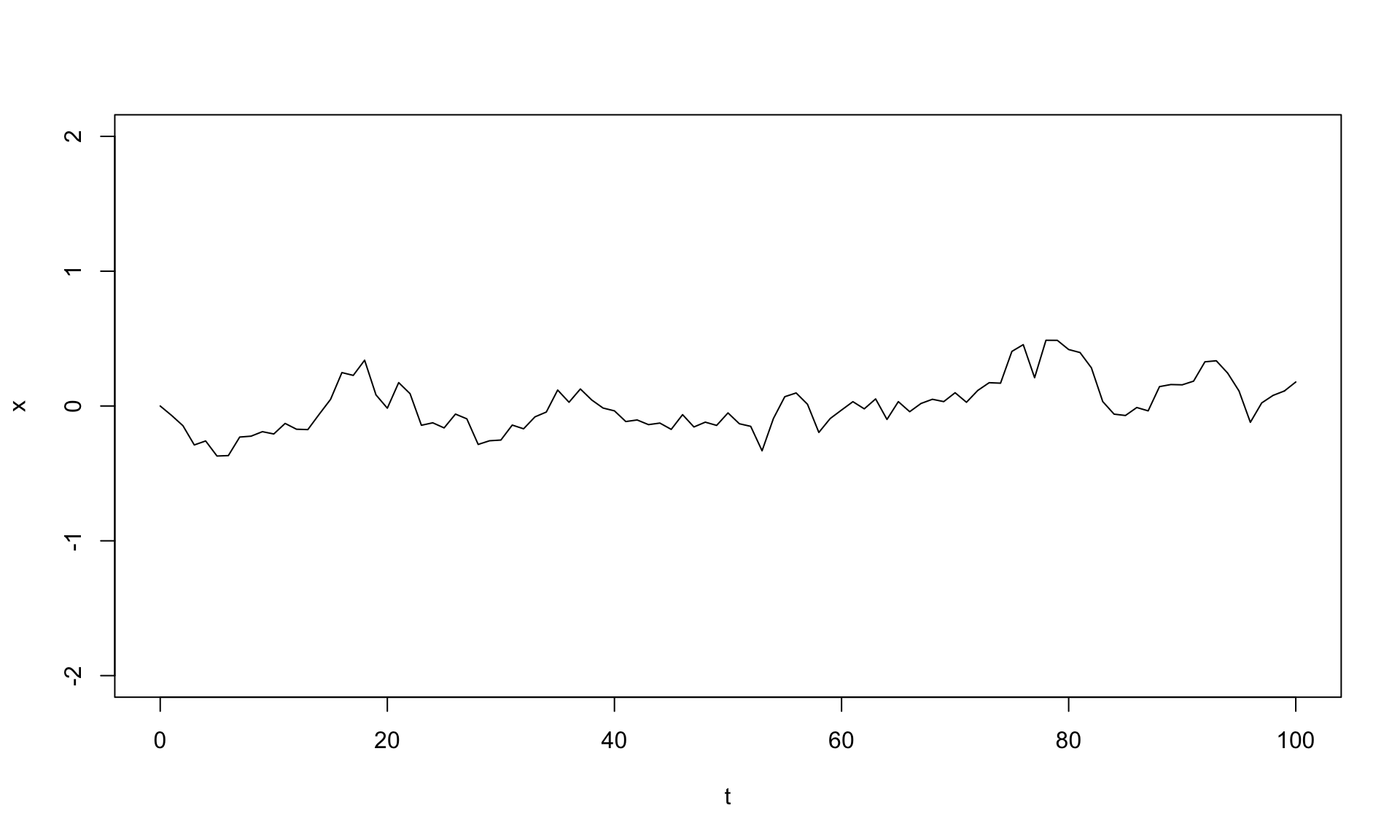## Exercise 3.1: Simulating Brownian motion in R

This short tutorial gives some simple approaches that can be used to simulate Brownian evolution in continuous and discrete time, in the absence of and on a phylogenetic tree.

Brownian motion is a stochastic continuous-time random walk model in which changes from one time to the next are random draws from some distribution with mean 0.0 and variance σ2. The expected variance under Brownian motion increases linearly through time with instantaneous rate σ2.

Brownian motion is very easy to simulate. To start off, let’s simulate a single instance of Brownian motion for 100 generations of discrete time in which the variance of the diffusion process is σ2 = 0.01 per generation. In this case, we will draw our evolutionary changes from a normal distribution; however it’s worth noting that (due to the CLT) regardless of the distribution, evolution will proceed by Brownian motion as the width of our timesteps decrease towards zero!

``````t<-0:100 # time
sig2<-0.01
## first, simulate a set of random deviates
x<-rnorm(n=length(t)-1,sd=sqrt(sig2))
## now compute their cumulative sum
x<-c(0,cumsum(x))
plot(t,x,type="l",ylim=c(-2,2))``````The trick here is that we draw random normal deviates for the change over each t time intervals; and then to get the state of our chain at each time interval we just compute the cumulative sum of all the individual changes using `cumsum`. We can do this because the distribution of the changes under Brownian motion is invariant and does not depend on the state of the chain.

We can also easily do a whole bunch of simulations like this at once, using the same conditions:

``````nsim<-100
X<-matrix(rnorm(n=nsim*(length(t)-1),sd=sqrt(sig2)),nsim,length(t)-1)
X<-cbind(rep(0,nsim),t(apply(X,1,cumsum)))
plot(t,X[1,],xlab="time",ylab="phenotype",ylim=c(-2,2),type="l")
apply(X[2:nsim,],1,function(x,t) lines(t,x),t=t)``````